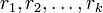# Classification of alternating groups having a class-inverting automorphism

This article classifies the members in a particular group family alternating group that satisfy the group property group having a class-inverting automorphism.

## Statement

The alternating group$A_n$ of degree$n$ is a group having a class-inverting automorphism (i.e., it has a class-inverting automorphism, an automorphism sending every element into the conjugacy class of its inverse) if and only if$n = 1,2,3,4,5,6,7,8,10,12,14$ (note that the case$n =1$ may not be considered alternating groups in some definitions).

## Facts used

1. Splitting criterion for conjugacy classes in the alternating group
2. Automorphism group of alternating group equals symmetric group when$n \ne 2,6$.

## Proof

### Overall plan

If$g \in A_n$ has the property that its conjugacy class in$S_n$ does not split in$A_n$, then$g$ is conjugate to$g^{-1}$ in$A_n$ (because they're conjugate in$S_n$). Thus, it suffices to check whether every element whose conjugacy class does split inside$A_n$, is conjugate to its inverse.

By the splitting criterion for conjugacy classes, it suffices to look at those even permutations that arise as products of cycles of distinct odd lengths. Further, in order to determine whether such a product of cycles is conjugate to its inverse in$A_n$, it suffices to find one permutation that conjugates this cycle to its inverse. If that one permutation is even, then the element is conjugate to its inverse. If that one permutation is odd, then the element is not conjugate to its inverse in$A_n$.

### Criterion for determining whether an element is conjugate to its inverse

For a cycle of odd length$(a_1, a_2, \dots, a_r)$, the product of$(r-1)/2$ transpositions$(a_j,a_{r+2-j})$ conjugates this cycle to its inverse. Thus, if a permutation is a product of cycles of odd lengths$r_1,r_2,\dots,r_k$, then there is a product of$\sum (r_i - 1)/2$ transpositions that conjugates this to its inverse.

The upshot: a product of cycles of distinct odd lengths$r_1,r_2,\dots,r_k$ is conjugate to its inverse if and only if$\sum (r_i - 1)/2$ is even. Equivalently, it is conjugate to its inverse if and only if the number of$r_i$s that are congruent to$3$ modulo$4$ is even.

### What we want

We have shown above that an element of$A_n$ whose conjugacy class splits in$A_n$ is conjugate to its inverse iff the number of cycle sizes that are congruent to$3$ mod$4$ is even. We now consider three cases for given$n$:

1. For all conjugacy classes that split in$A_n$, the number of cycle sizes that are congruent to$3$ mod$4$ is even: In this case, every element is conjugate to its inverse, the group is an ambivalent group, and the identity map is a class-inverting automorphism.
2. For all conjugacy classes that split in$A_n$, the number of cycle sizes that are congruent to$3$ mod$4$ is odd: In this case, no element whose conjugacy class splits is conjugate to its inverse in$A_n$. Thus, conjugating by any odd permutation (an outer automorphism of$A_n$) sends every such element into the conjugacy class of its inverse. The elements whose conjugacy class does not split in$A_n$ anyway get sent to the conjugacy class of their inverse. Thus, conjugation by any odd permutation is a class-inverting automorphism.
3. Of the conjugacy classes that split, there is at least one with an even number of cycle sizes that are congruent to$3$ mod$4$ and at least one with an odd number of cycle sizes that are congruent to$3$ mod$4$. In this case, neither conjugation by an even permutation nor conjugation by an odd permutation is class-inverting. Thus, no conjugation by an element in the symmetric group is class-inverting. But by fact (2), unless$n = 2,6$, these are all the automorphisms, so the group has no class-inverting automorphism.

The upshot is that if cases (1) or (2) hold, there is a class-inverting automorphism. If case (3) holds and$n ne 2,6$, there is no class-inverting automorphism. (It turns out that both$n=2$ and$n=6$ fall under case (1), so this is not an issue).

### Testing for different$n$

• If$n = 9 + 4k, k \ge 0$, then case (3) holds. In particular, this applies for$n = 9,13,17,\dots$: If$n = 9 + 4k$, then$n = (n-4) + 3 + 1$, a partition with distinct odd parts, an odd number of which is$3$ mod$4$. On the other hand,$n = n$, a partition with distinct odd parts, an even number of which are$3$ mod$4$.
• If$n = 11 + 4k, k \ge 0$, then case (3) holds. In particular, this applies for$n = 11,15,19,\dots$: If$n = 11 + 4k$, then$n = (n-4) + 3 + 1$, a partition with distinct odd parts, an even number of which is$3$ mod$4$. On the other hand,$n = n$, a partition with distinct odd parts, an odd number of which are$3$ mod$4$.
• If$n = 16 + 4k, k \ge 0$, then case (3) holds. In particular, this applies for$n = 16,20,\dots$: If$n = 16 + 4k$, then$n = (n - 9) + 5 + 3 + 1$, a partition with distinct odd parts, an even number of which are$3$ mod$4$. On the other hand,$n = (n-5) + 5$, a partition with distinct odd parts, an odd number of which are$3$ mod$4$.
• If$n = 18 + 4k, k \ge 0$, then case (3) holds. In particular, this applies for$n = 18,22,\dots$: If$n = 18 + 4k$, then$n = (n - 9) + 5 + 3 + 1$, a partition with distinct odd parts, an odd number of which are$3$ mod$4$. On the other hand,$n = (n-5) + 5$, a partition with distinct odd parts, an even number of which are$3$ mod$4$.

This leaves out only the possibilities$n = 1,2,3,4,5,6,7,8,10,12,14$. Case (1) applies to$n = 1,2,5,6,10,14$ (these are the ambivalent groups, see also classification of ambivalent alternating groups) and case (2) applies to$n = 3,4,7,8,12$.